# 是哪位高人琢磨出的这条微信？太牛了 !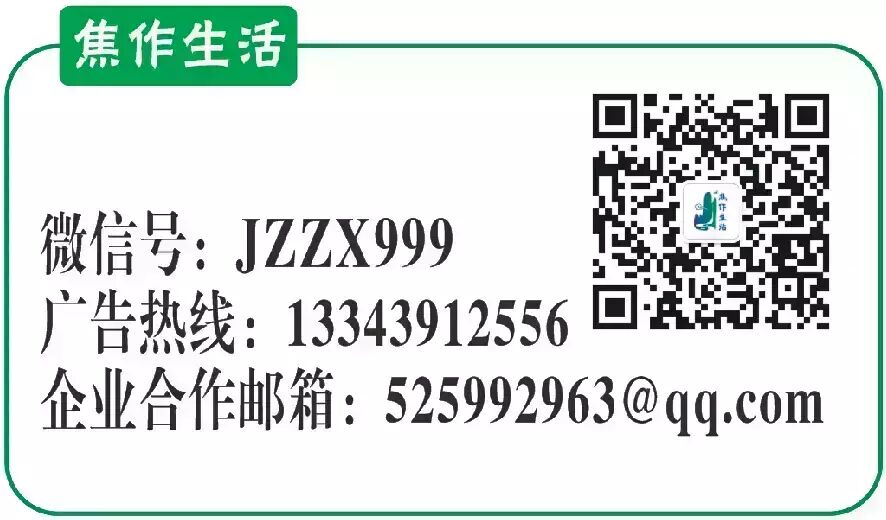1 2 3 4 5 6 7 8 9 10 11 12 13 14 1516 17 18 19 20 21 22 23 24 25 26。那么：

Knowledge (知识)： K+N+O+W+L+E+D+G+E＝ 11+14+15+23+12+5+4+7+5=96%。

Workhard (努力工作）：W+O+R+K+H+A+R+D＝ 23+15+18+11+8+1+18+4 =98%。

Luck（好运） L+U+C+K＝12+21+3+11=47%。

Love（爱情） L+O+V+E＝12+15+22+5=54%。

L+E+A+D+E+R+S+H+I+P=12+5+1+4+5+18+19+9+16=89%

ATTITUDE (心态) A+T+T+I+T+U+D+E＝1+20+20+9+20+21+4+5=100%。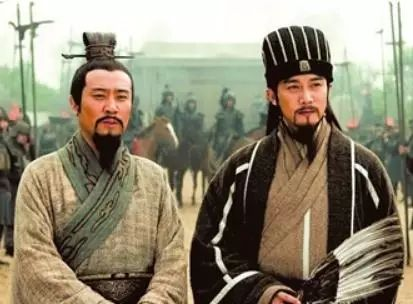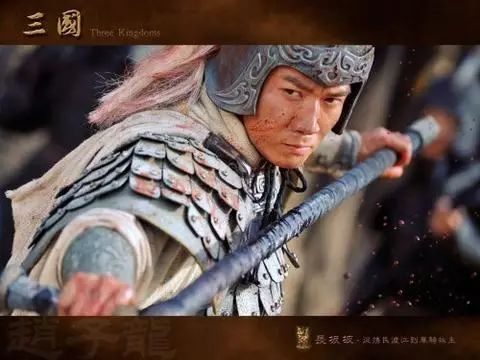——如若万事俱备 你的价值何在！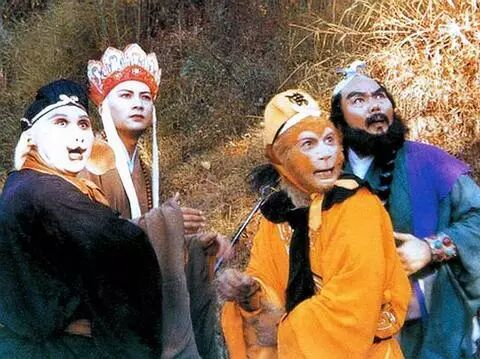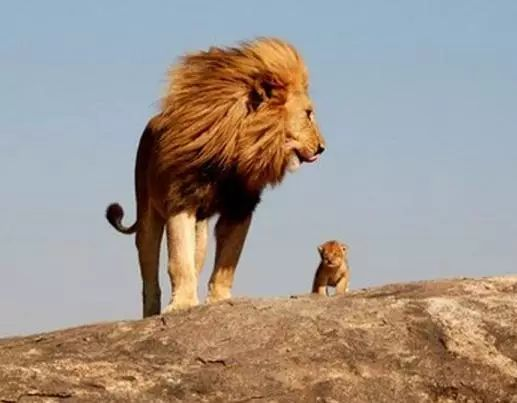"让疯狗咬一口倒霉不？"

——不是什么人都配做你的对手，不要与那些没有素质的人争辩，微微一笑远离他，不要让他咬到你。2016年，记住这励志正能量的话语！送给所有孜孜不倦努力着的人!